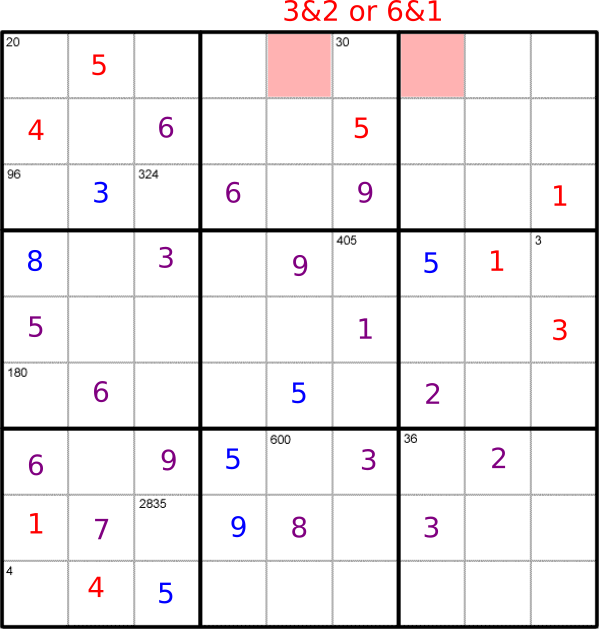#### You may also likeMake a set of numbers that use all the digits from 1 to 9, once and once only. Add them up. The result is divisible by 9. Add each of the digits in the new number. What is their sum? Now try some other possibilities for yourself!### N000ughty Thoughts

How many noughts are at the end of these giant numbers?### DOTS Division

Take any pair of two digit numbers x=ab and y=cd where, without loss of generality, ab > cd . Form two 4 digit numbers r=abcd and s=cdab and calculate: {r^2 - s^2} /{x^2 - y^2}.

# Product Sudoku

##### Age 11 to 16Challenge Level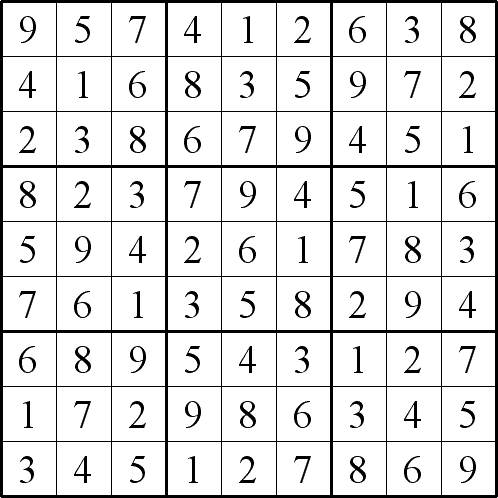Amelia approached the problem like this. Click on the images to see a larger version.

First, I used the smaller numbers that there aren't many ways to make using the digits 1 to 9.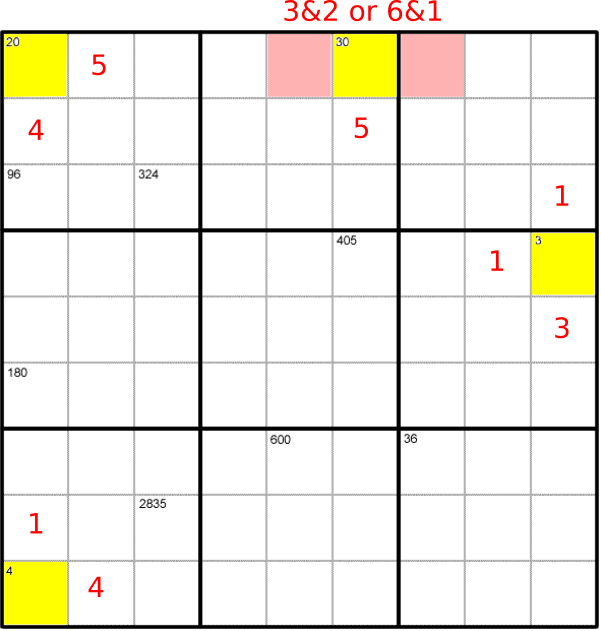Then I used the numbers that are so big that there aren't many way to make them using the numbers 1 to 9.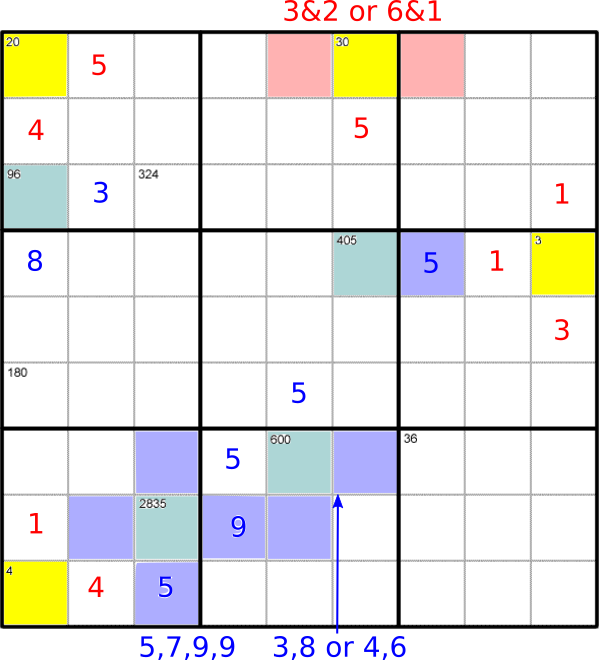Then I used the other numbers. I had to use lots of the rules of sudoku as well.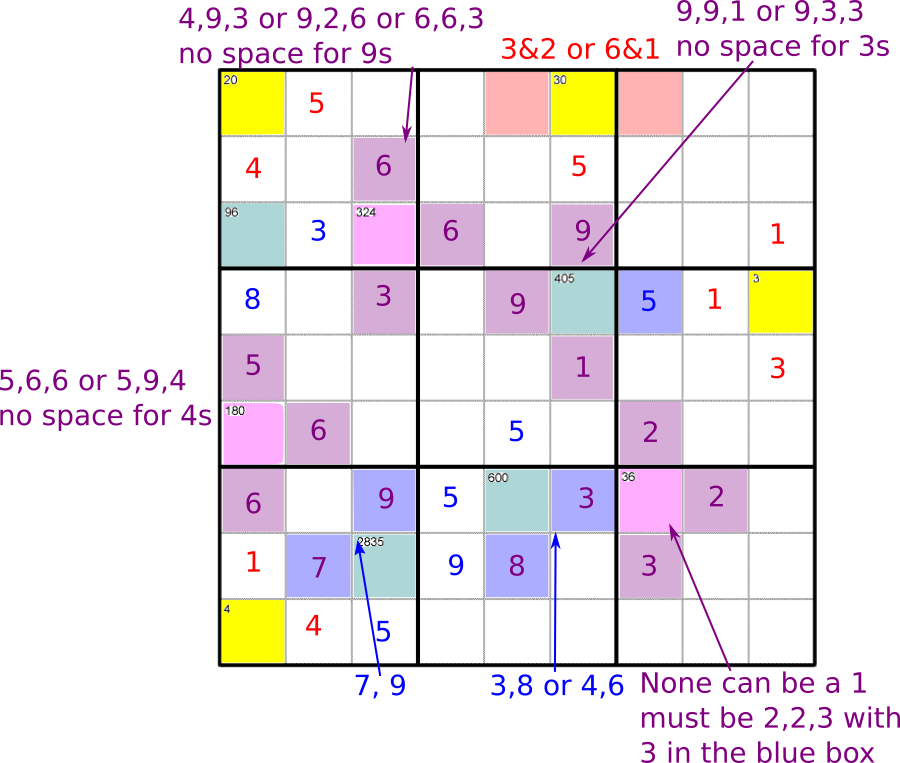After that, I could finish it like a normal sudoku.